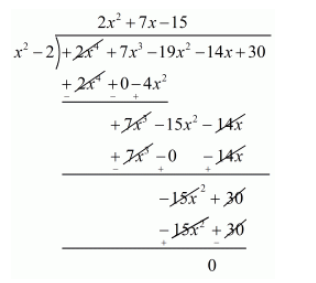# Find all zeros of the polynomial 2x4 + 7x3 − 19x2 − 14x + 30,

Question:

Find all zeros of the polynomial $2 x^{4}+7 x^{3}-19 x^{2}-14 x+30$, if two of its zeros are $\sqrt{2}$ and $-\sqrt{2}$.

Solution:

We know that if $x=\alpha$ is a zero of a polynomial, and then $x-\alpha$ is a factor of $f(x)$.

Since $\sqrt{2}$ and $-\sqrt{2}$ are zeros of $f(x)$.

Therefore

$(x+\sqrt{2})(x-\sqrt{2})=x^{2}-(\sqrt{2})^{2}$

$=x^{2}-2$

$x^{2}-2$ is a factor of $f(x)$. Now, we divide $2 x^{4}+7 x^{3}-19 x^{2}-14 x+30$ by $g(x)=x^{2}-2$ to find the zero of $f(x)$.By using division algorithm we have $f(x)=g(x) \times q(x)-r(x)$

$2 x^{4}+7 x^{3}-19 x^{2}-14 x+30=\left(x^{2}-2\right)\left(2 x^{2}+7 x-15\right)+0$

$2 x^{4}+7 x^{3}-19 x^{2}-14 x+30=(x+\sqrt{2})(x-\sqrt{2})\left(2 x^{2}+10 x-3 x-15\right)$

$2 x^{4}+7 x^{3}-19 x^{2}-14 x+30=(x+\sqrt{2})(x-\sqrt{2})[2 x(x+5)-3(x+5)]$

$2 x^{4}+7 x^{3}-19 x^{2}-14 x+30=(x+\sqrt{2})(x-\sqrt{2})(2 x-3)(x+5)$

Hence, the zeros of the given polynomial are $-\sqrt{2},+\sqrt{2}, \frac{+3}{2},-5$.